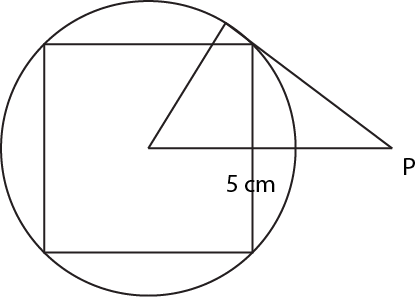Question 40

# A circle circumscribes a square. What is the area of the square?I. Radius of the circle is given.II. Length of the tangent from a point 5 cm away from the centre of the circle is given.

Solution

As the circle circumscribes the square, diameter of the circle= diagonal of the square. Let the side of the circle be s. If we can determine the value of s, then we can find the area of the square $$s^2$$.

Statement 1: Let the radius be r. 2r = $$s \sqrt{2}$$. Hence, s = $$r\sqrt{2}$$. Thus, we can find the area of the circle using this information.

Statement 2: This scenario can be drawn as shown below:A line drawn from the center of the circle intersects the tangent at an angle of 90°. Hence, the triangle formed is a right-angled triangle with the hypotenuse of 5 cm, one side equal to the radius of the circle and second side equal to the length of the tangent. If the length of the tangent is known then we can calculate the radius using Pythogoras theorem. If the radius is known, the area of the square can be calculated as shown above.